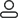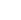LanguageLogin|Sign up
Blog >> Blog Details Page
Engineer Summary: Signal Integrity Design Experience 100 (1)
Posted:02:54 PM August 07, 2018 writer: G

1. The signal rise time is about 10% of the clock cycle, which is 1/10x1/Fclock. For example, the rise time in 100MHZ is about 1NS.

2. The amplitude of the Nth harmonic of the ideal square wave is about 2/(N pie) times the secondary value of the clock voltage. For example, the first harmonic amplitude of the 1V clock signal is approximately 0.6V, and the amplitude of the third harmonic is approximately 0.2V.

3. The relationship between the bandwidth of the signal and the rise time is: BW=0.35/RT. For example, if the rise time is 1 NS, the bandwidth is 350 MHz. If the bandwidth of the interconnect is 3 GHz, the minimum rise time it can transmit is about 0.1 NS.

4. If you do not know the rise time, you can think that the signal bandwidth is about 5 times the clock frequency.

5. The resonant frequency of the LC circuit is 5 GHz/sqrt (LC), the unit of L is NH, and the unit of C is PF.

6. In 400MHZ, the axial pin resistance can be regarded as an ideal resistance; within 2GHZ, the SMT0603 resistor can be regarded as an ideal resistor.

7. The ESL (pin resistance) of the axial pin resistance is approximately 8 NH, and the ESL of the SMT resistor is approximately 1.5 NH.

8. The unit length resistance of a near-bond wire with a diameter of 1 mil is approximately 1 ohm/IN.

9, 24AWG wire diameter is about 20MIL, resistivity is about 25 milliohms / FT.

The square resistivity of a 10 ounce barrel line is approximately 0.5 ohms per square.

11. At 10MHZ, a 1 ounce copper line begins to have a skin effect.

12. The capacitance of a 1IN sphere is approximately 2PF.

13. A coin-sized pair of parallel plates. When the plates are filled with air, the capacitance between them is about 1 PF.

14. When the distance between the plates of the capacitors is equal to the width of the board, the capacitance generated by the edges is equal to the capacitance generated by the parallel plates. For example, when estimating the parallel plate capacitance of a microstrip line with a line width of 10 mil and a medium thickness of 10 mil, the estimated value is 1 PF/IN, but the actual capacitance is about twice the above, that is, 2 PF/IN.

15. If you do not know anything about the material properties, only know that it is an organic insulator, it is considered to have a dielectric constant of about 4.

16. A chip with a power of 1W. The decoupling capacitor (F) can provide a charge so that the voltage drop is less than 5% (S) is C/2.

17. In a typical board clock, when the dielectric thickness is 10 mil, the coupling capacitance between the power supply and the ground plane is 100 PF/IN square, and it is inversely proportional to the thickness of the medium.

18. If the 50 ohm microstrip line has a bulk dielectric constant of 4, its effective dielectric constant is 3.

19. The local inductance of a 1 MIL round wire is approximately 25 NH/IN or 1 NH/MM.

20. A circular coil of 1IN in diameter made up of 10 mil thick lines. Its size is equivalent to the thumb and forefinger, and its loop inductance is about 85 NH.

21. The inductance per unit length of a ring with a diameter of 1IN is approximately 25 NH/IN or 1 NH/MM. For example, if the package lead is part of a loop wire and is 0.5IN long, its inductance is about 12NH.

22. When the center distance of a pair of round bars is less than 10% of their respective lengths, the local mutual inductance is about 50% of the respective local mutual inductance.

23. When the center distance of a pair of round bars is equal to their own length, the local mutual inductance between them is less than 10% of their respective local mutual inductance.

24. The loop inductance of SMT capacitors (including surface wiring, vias, and capacitors themselves) is approximately 2NH. To reduce this value below 1NH requires a lot of work.

25. The loop inductance per unit area of the plane is 33 PHx dielectric thickness (MIL).

26. The larger the diameter of the via, the lower its diffusion inductance. A diffused inductor with a 25 mil diameter via is approximately 50 PH.

27. If there is a sand hole area, when the free area accounts for 50%, the loop inductance between the plane pairs will increase by 25%.

28. The skin depth of copper is inversely proportional to the square of the frequency. At 1 GHz, it is 2 UM. Therefore, at 10 MHz, the skin of copper is 20 UM.

29. In a 50 ohm 1 ounce copper transmission line, the loop inductance per unit length is a constant when the frequency is above about 50 MHz. This means that the characteristic impedance is constant at frequencies above 50 MHz.

30. The speed of electrons in copper is extremely slow, which is equivalent to the speed of ants, which is 1CM/S.

31. The speed of the signal in the air is about 12IN/NS. The signal speed in most polymeric materials is about 6IN/NS.

32. In most rolling materials, the line delay 1/V is about 170PS/IN.

33. The spatial extension of the signal is equal to the rise time X speed, ie RTx6IN/NS.

34. The characteristic impedance of the transmission line is inversely proportional to the capacitance per unit length.

35. In FR4, the capacitance per unit length of all 50 ohm transmission lines is approximately 3.3 PF/IN.

36. In FR4, the inductance per unit length of all 50 ohm transmission lines is approximately 8.3 NH/IN.

37. For a 50 ohm microstrip line in FR4, the dielectric thickness is approximately half the line width.

38. For the 50 ohm stripline in FR4, the spacing between planes is twice the width of the signal line.

39. The impedance of the transmission line is the characteristic impedance within much less than the return time of the signal. For example, when driving a 3 Ω long 50 ohm transmission line, all the rise time is short and the 1 NS drive source is traveling along the line and the rise time is felt to be a 50 ohm constant load.

40. The relationship between the total capacitance and the delay of a transmission line is C=TD/Z0.

41. The relationship between the total loop inductance and the delay of a transmission line is L=TDxZ0.

42. If the return path width in the 50 ohm microstrip line is equal to the signal line width, the characteristic impedance is 20% higher than the characteristic impedance when the return path is infinitely wide.

43. If the return path width in the 50 ohm microstrip line is at least 3 times the signal line width, the characteristic impedance is less than 1% deviation from the characteristic impedance when the return path is infinite.

44, the thickness of the wiring can affect the characteristic impedance, the thickness is increased by 1 MIL, the impedance is reduced by 2 ohms.

45. The solder mask thickness of the microstrip line will reduce the characteristic impedance, increase the thickness by 1 MIL, and reduce the impedance by 2 ohms.

46. In order to obtain an accurate lumped circuit approximation, at least 3.5 LC sections are required in the spatial extension of each rise time.

47. The bandwidth of the single-cell LC model is 0.1/TD.

48. If the transmission line delay is shorter than 20% of the signal rise time, there is no need to terminate the transmission line.

49. In a 50 ohm system, the reflection coefficient due to a 5 ohm impedance change is 5%.

50. Keep all mutations (IN) as short as possible for the rise time (NS).

584 1 0 1
• PCB
Prototype
• PCB
Assembly
• SMD
Stencil
 Dimensions: (mm) × Quantity: (pcs) 5 5 10 15 20 25 30 40 50 75 100 120 150 200 250 300 350 400 450 500 600 700 800 900 1000 1500 2000 2500 3000 3500 4000 4500 5000 5500 6000 6500 7000 7500 8000 9000 10000 Other Quantities:(quantity*length*width is greater than 10㎡) OK Layers: 1 2 4 6 8 10 12 Thickness: 0.6 mm 0.8 mm 1.0 mm 1.2 mm 1.6 mm 2.0 mm 2.5 mmQuote now